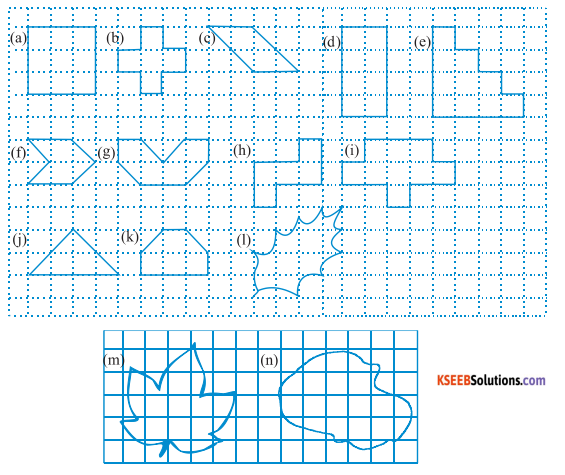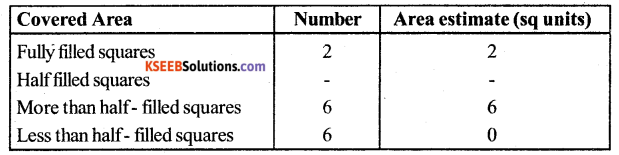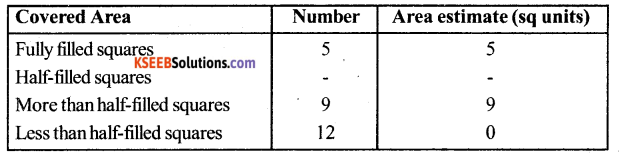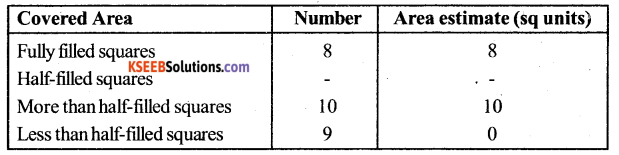# KSEEB Solutions for Class 6 Maths Chapter 10 Mensuration Ex 10.2

Students can Download Chapter 10 Mensuration Ex 10.2 Questions and Answers, Notes Pdf, KSEEB Solutions for Class 6 Maths helps you to revise the complete Karnataka State Board Syllabus and score more marks in your examinations.

## Karnataka State Syllabus Class 6 Maths Chapter 10 Mensuration Ex 10.2

Question 1.
Find the areas of the following figures by counting squareSolution:
(a) The figure contains 9 fully filled squares only. Therefore, the area of this figure will be 9 square units.
(b) The figure contains 5 fully filled squares only. Therefore, the area of this figure will be 5 square units.
(c) The figure contains 2 fully filled squares and 4 half-filled squares. Therefore, the area of this
figure will be 4 square units.
(d) The figure contains 8 fully filled squares only. Therefore, the area of this figure will be 8 square units.
(e) The figure contains 10 fully filled squares only. Therefore, the area of this figure will be 10 square units.
(f) The figure contains 2 fully filled squares and 4 half-filled squares. Therefore, the area of this figure will be 4 square units.
(g) The figure contains 4 fully filled squares and 4 half-filled squares. Therefore, the area of this figure will be 6 square units.
(h) The figure contains 5 fully filled squares only. Therefore, the area of this figure will be 5 square units.
(i) The figure contains 9 fully filled squares only. Therefore, the area of this figure will be 9 square units.
(j) The figure contains 2 fully filled squares and 4 half-filled squares. Therefore, the area of this figure will be 4 square units.
(k) The figure contains 4 fully filled squares and 2 half-filled squares. Therefore, the area of this figure will be 5 square units.
(l) From the given figure, it can be observed that,Total area = 2 + 6 = 8 square units(m) From the given figure, it can be observed that,Total area = 5 + 9 = 14 square units
(n) From the given figure, it can be observed that,Total area = 8 + 10 = 18 square units